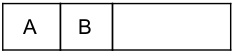Graphs-and-Tree

 Question 1
Consider the following statements:
(a) Depth first search is used to traverse a rooted tree.
(b) Preorder, Postorder and Inorder are used to list the vertices of an ordered rooted tree.
(c) Huffman’s algorithm is used to find an optimal binary tree with given weights.
(d) Topological sorting provides a labelling such that the parents have larger labels than their children.
Which of the above statements are true?
 A (a) and (b) B (c) and (d) C (a), (b) and (c) D (a), (b), (c) and (d)
Data-Structures       Graphs-and-Tree       UGC NET CS 2015 Jun- paper-2
Question 1 Explanation:
→ Preorder, Postorder and Inorder are traversing techniques where all the nodes of the tree will be traversed from the root node.
→ Depth-first search (DFS) is an algorithm for traversing or searching tree or graph data structures. The algorithm starts at the root node (selecting some arbitrary node as the root node in the case of a graph) and explores as far as possible along each branch before backtracking.
→ The optimal binary tree, which has minimum weighted path length when the characters of the alphabet are stored at its n terminal nodes.An optimal binary tree generates what is called a Huffman code
→ A topological sort or topological ordering of a directed graph is a linear ordering of its vertices such that for every directed edge uv from vertex u to vertex v, u comes before v in the ordering. For instance, the vertices of the graph may represent tasks to be performed, and the edges may represent constraints that one task must be performed before another; in this application, a topological ordering is just a valid sequence for the tasks.
A topological ordering is possible if and only if the graph has no directed cycles, that is, if it is a directed acyclic graph (DAG). Any DAG has at least one topological ordering, and algorithms are known for constructing a topological ordering of any DAG in linear time.
 Question 2
Level order Traversal of a rooted Tree can be done by starting from root and performing:
 A Breadth First Search B Depth First Search C Root Search D Deep Search
Data-Structures       Graphs-and-Tree       UGC NET CS 2015 Jun- paper-2
Question 2 Explanation:
It is an algorithm for traversing (or) searching tree (or) graph data structures. It starts at the root and explores all of the neighbour nodes at the present depth prior to moving on to the nodes at the next depth level.
 Question 3
Weighted graph :
 A Is a bi-directional graph B Is directed graph C Is graph in which number associated with arc D Eliminates table method
Data-Structures       Graphs-and-Tree       UGC NET CS 2004 Dec-Paper-2
Question 3 Explanation:
→ Weighted graph is a graph in which number associated with arc. A weighted graph is a graph in which each branch is given a numerical weight.
→ A weighted graph is therefore a special type of labeled graph in which the labels are numbers.
 Question 4
Consider the graph, which of the following is a valid topological sorting ?A ABCD B BACD C BADC D ABDC
Data-Structures       Graphs-and-Tree       UGC NET CS 2005 Dec-Paper-2
Question 4 Explanation:
Finding valid topological sorting we have two methods.
1. Using DFS method
2. Using indegree elimination method
The simplest method is indegree elimination method.
Indegrees:
A →0
B →1
D →2
C → 1 (Final)
We can assume that indegree value of A is ‘0’. So, it is starting point.
Step-1: Remove ‘A’ from graph, then indegrees are
B →0
D →1
C →1
Sequence:Step-2: Remove ‘B’ from graph, then indegrees are
D →0
C →1
Sequence:Step-3: Remove ‘C’ from graph then indegrees are
C →0
Sequence:Question 5
Depth ion travels of the following directed graph is :A A B C D E F B A B D E F C C A C E B D F D None of the above
Data-Structures       Graphs-and-Tree       UGC NET CS 2007 June-Paper-2
Question 5 Explanation:
To find depth ion traverse using DFS.
Option-A: It is ruled out because we can’t move after C to D. It is violating DFS property.
Option-B: It is following actual DFS structure.Option-C: It is ruled out because after visiting E we can’t visit B again. It is violating DFS properties.
 Question 6
Pre order is also known as :
 A Depth first order B Breadth first order C Topological order D Linear order
Data-Structures       Graphs-and-Tree       UGC NET CS 2007 June-Paper-2
Question 6 Explanation:
→ Pre order is also known as depth first order.
→ A depth-first order is defined to be the reverse of the order in which we last visit the nodes of the control graph when we create the Depth first spanning tree(DFST).
There are 6 questions to complete.
PHP Code Snippets Powered By : XYZScripts.com